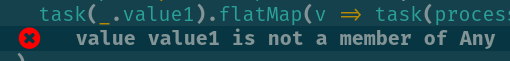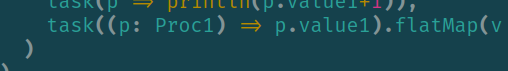# DSL and type inference

Hi im trying to make a simple dsl where I have these 2 entities Process and Step.

So far so good but I also need an Out type for each step.

The problem is I can’t make the flatMap function on Step because the [A] doesn’t get inferred. I’ve tried with context functions too so i can carry the [A] paramter over but it doesn’t work with flatmap either. Any clues?

It says `value1` is not a member of `Any`:so you need to provide the type for the anonymous function input to `task` instead of using the `_.value1` syntactic sugar:It seems to work now. Scastie - An interactive playground for Scala.

Thanks for the answer I’ve considered that too but I was looking for a way to let the compiler infer it somehow so it’s easier to write

To me it looks like the inference problem is unrelated to the additional `Out` parameter (and “dropping” it in `#sequence`, as I initially assumed), but can be reduced to the following:

[EDIT: Replaced `Proc1` with `String`.]

``````trait Step[A]:
def id: Step[A] = this

def id[A](s: Step[A]): Step[A] = s

def task[A](fn: A => Any): Step[A] = ???

// compiles
// fails: value length is not a member of Any
``````

So it looks like type inference handles function nesting, but not method chaining in this scenario…?I don’t know anything about compiler internals and constraints, but naively I would have expected this to work.

Thanks for identify the actual problem, I could try to avoid method call somehow but it would be easier if it worked.
Edit: actually method call isn’t the only problem probably. look this

``````trait Step[A]:
def id: Step[A] = this

def id[A](s: Step[A]): Step[A] = s

def sequence[A](s: Step[A])(s2: Step[A]): Step[A] = s
def ifThenElse[A](cond: A => Boolean, thenS: Step[A], elseS: Step[A]): Step[A] = ???

def task[A](fn: A => Any): Step[A] = ???

// compiles
Looks like the more you nest the more you lose inference